Physics
Fox

### Summary

• A parallel circuit is when the current goes along multiple paths: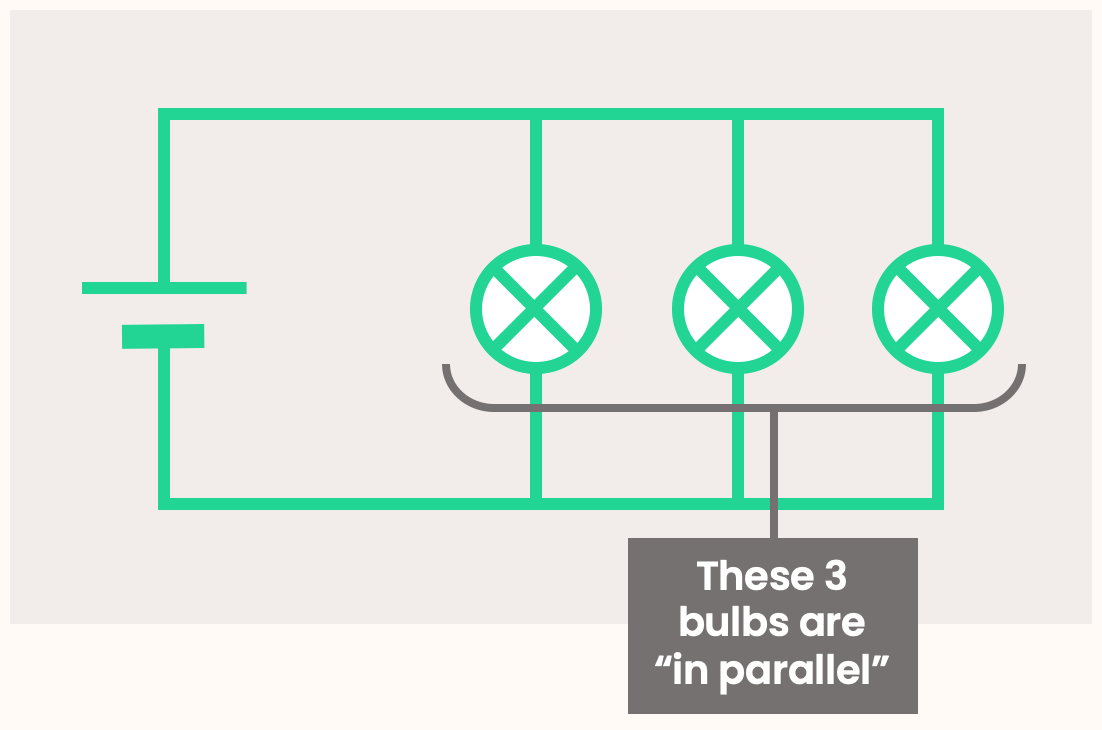• Components on neighbouring paths are said to be “in parallel”.

• The potential difference across each component is the same. It is also the same as the voltage of the battery.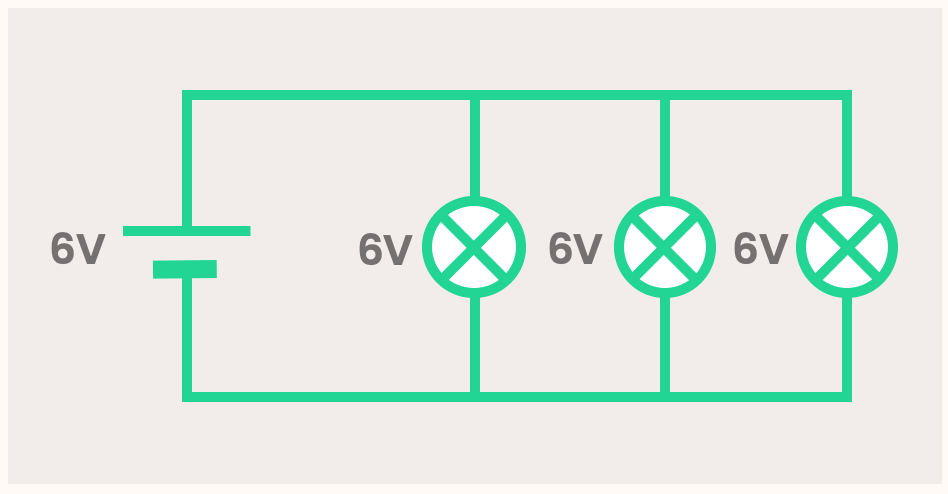• When a wire splits into many paths, the current is shared between these paths. Therefore, the current through each component adds up to the current leaving the battery. The current might not be shared equally!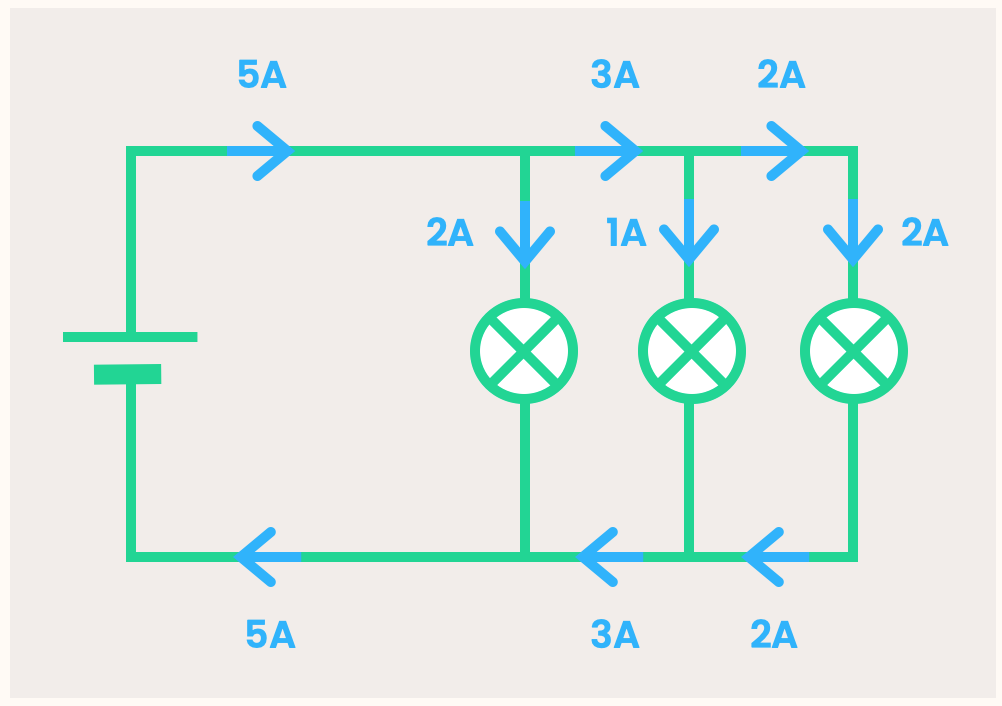Let's look at the water circuit again.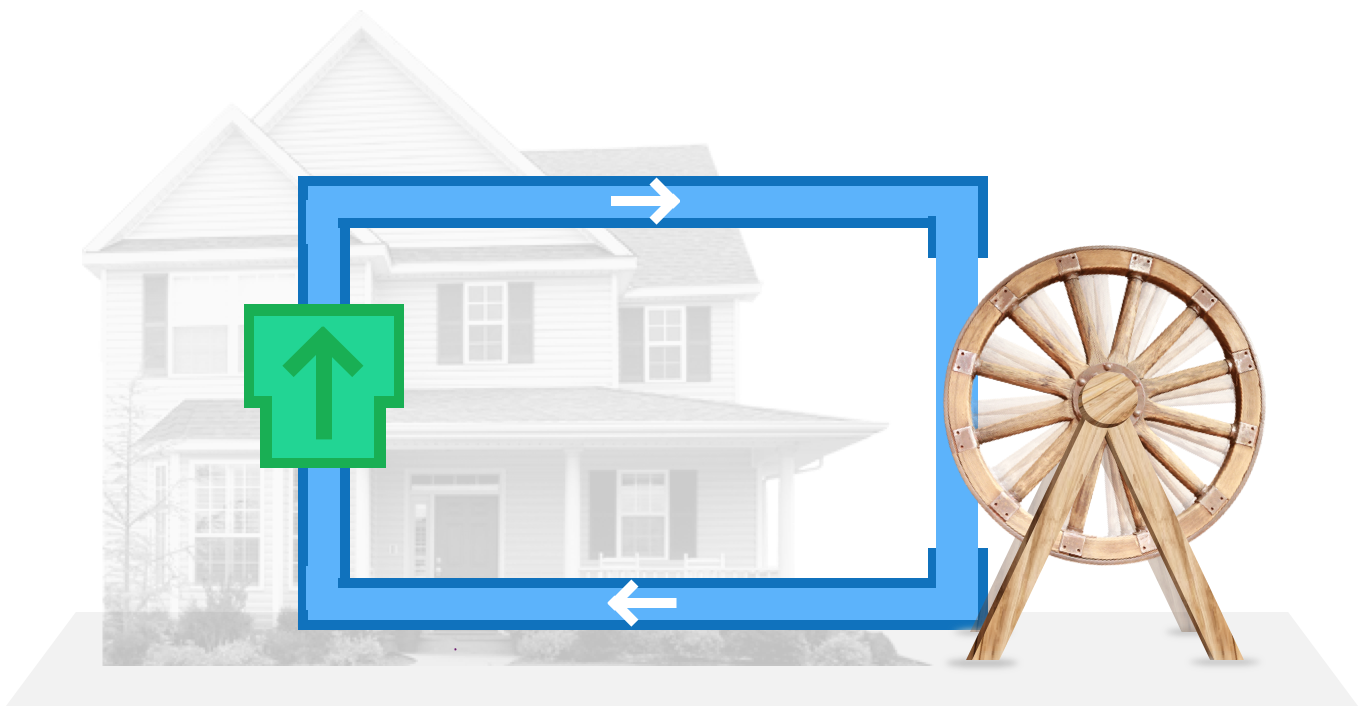As always, the pump takes the water to a higher potential energy. This energy is released when it comes back down on the right, and is used to power the waterwheel.

Let's add another waterwheel. Unlike with a series circuit, let's put the new waterwheel on a separate path to the old one: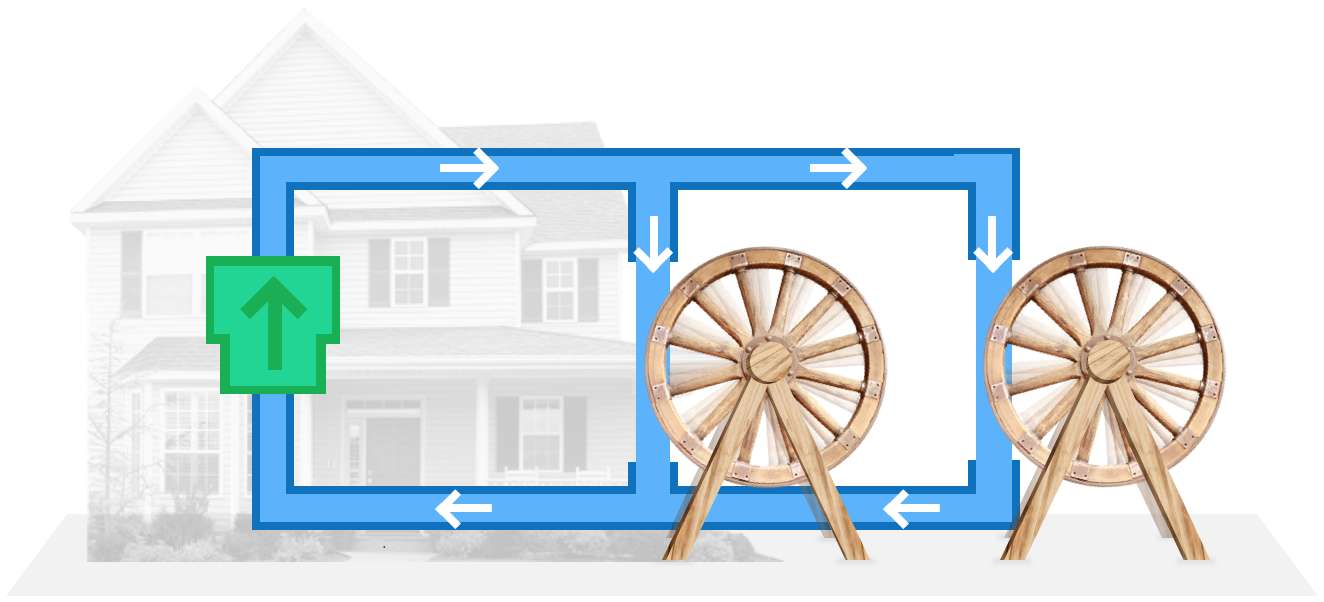When the current goes along multiple paths, it is called a parallel circuit.

Because the pipe splits into two, this means the current must be shared between the each pipe.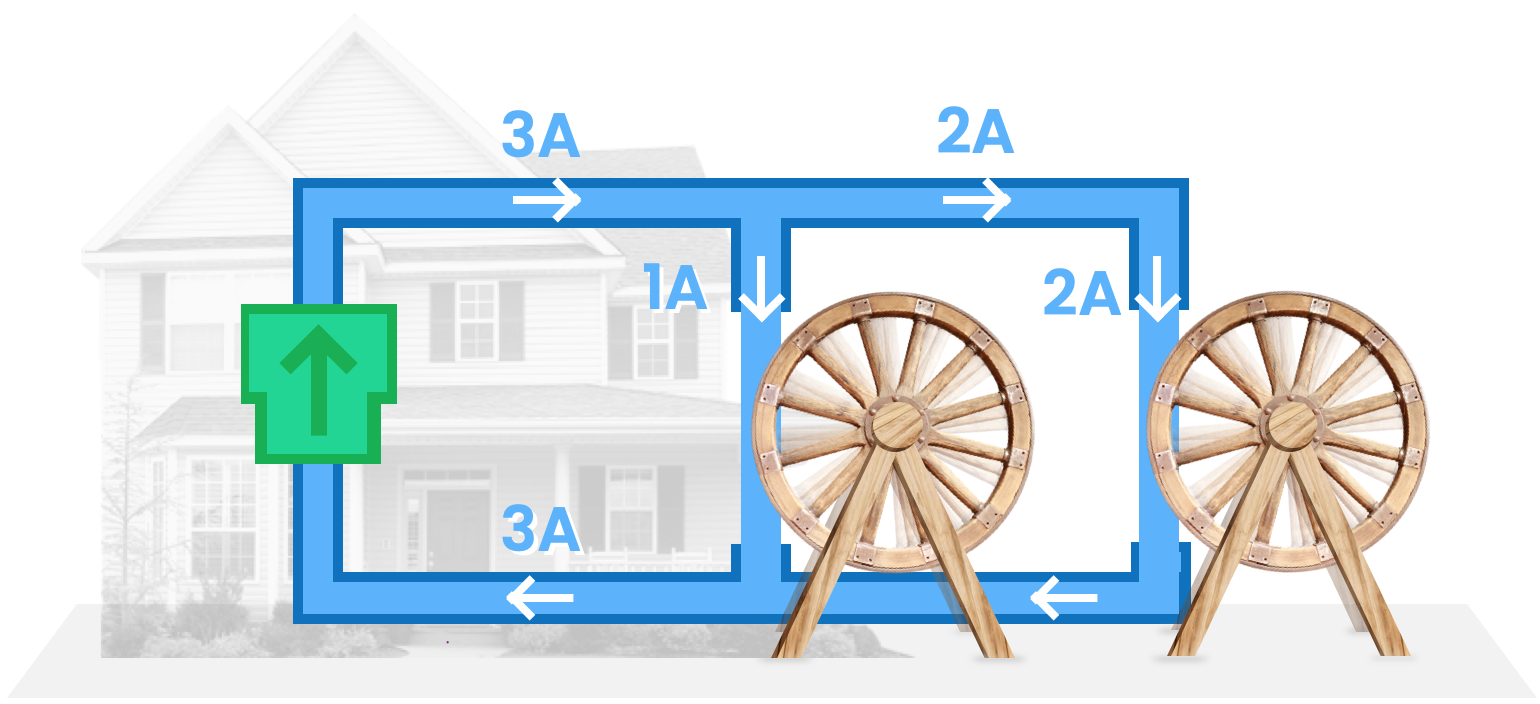When a pipe splits, the current must be shared between each pipe. In this example, a third of the water flows one way, and two-thirds of the water flows the other way.

This is true however many new paths we add. So let's add another one: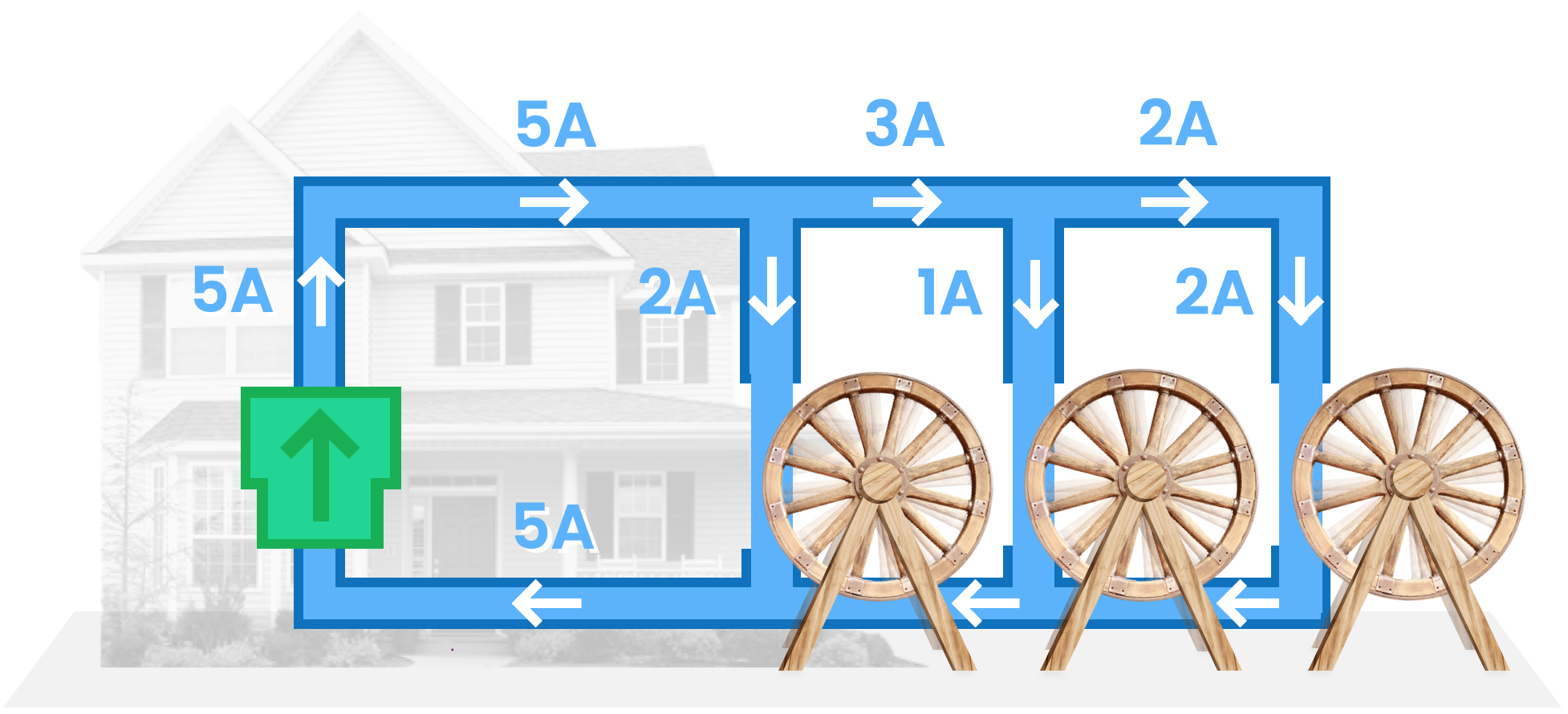Whenever a pipe splits, the current must be shared between each pipe. Note how the current flowing through each waterwheel adds up to the current flowing through the pump. (2A + 1A + 2A = 5A)

Note that the potential energy of the water does not change each time the pipe splits (because it's still on the roof). The potential energy of the water only decreases when it goes down. This means the potential difference (i.e. difference in P.E. for each water molecule) is the same across each waterwheel (since its dropping the same height for each one).

As always, what's true for the water circuit is also true for the electrical circuit.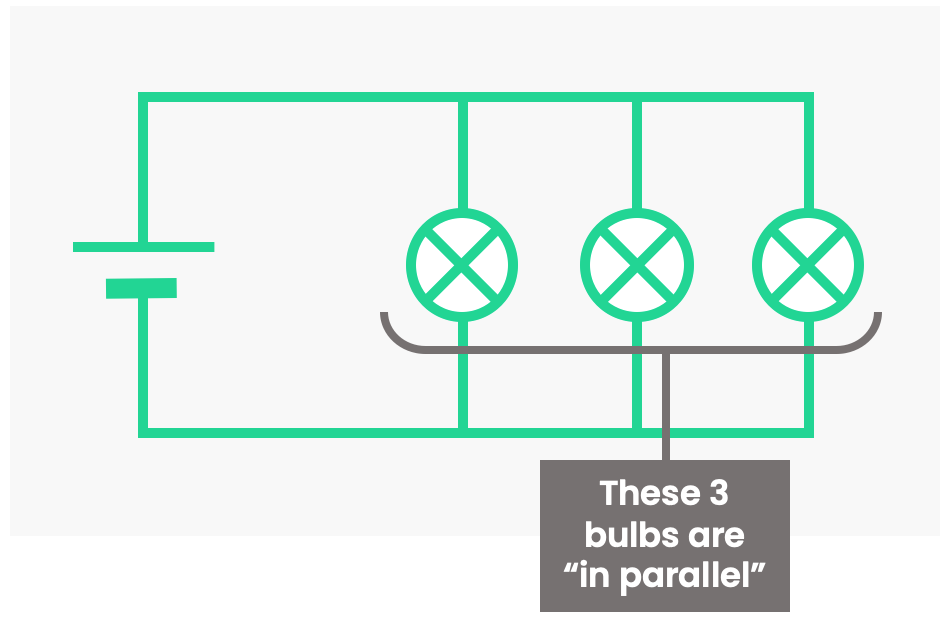This circuit is a parallel circuit because the current goes along multiple paths. Components on neighbouring paths are said to be “in parallel”. A way to remember this: Paths which are in parallel are drawn as parallel lines.

Much like the water circuit, whenever a wire splits in two, this means the current must be shared between the each wire (NB: It might not be shared equally). A result of this: the current through each component adds up to the current leaving the battery.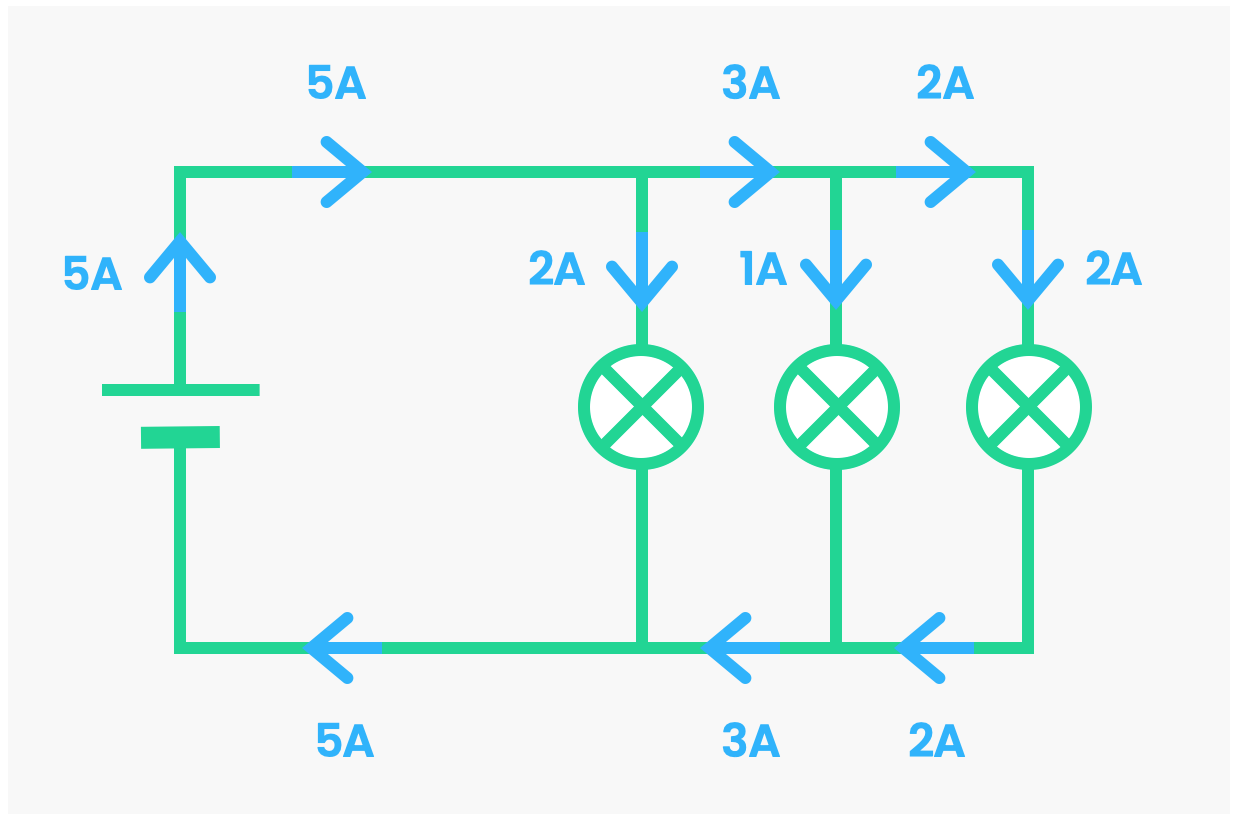Current splits in a parallel circuit. As a result, the current though each component adds up to the current through the cell (5A = 2A + 1A + 2A).

Note that the potential energy of the electrons does not change each time the path splits. The potential energy of electrons can only decrease when going through a component. This means the potential difference (i.e. difference in P.E. for each electron) is the same across each component.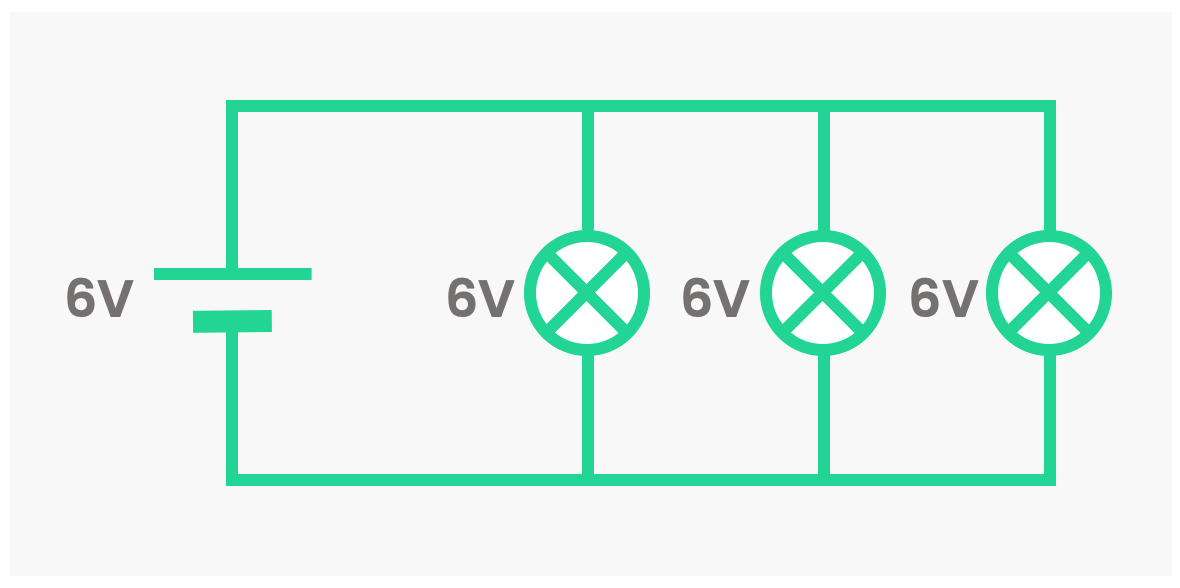An important advantage of parallel circuits is that they still work if one component in them breaks.

Let's say you have some Christmas lights, and they're all wired in series. What happens if one bulb breaks? Suddenly, none of the lights will be on, as the circuit is now incomplete.Series: If one bulb breaks, no current can flow

Now let's wire them up in parallel. If one breaks, all the others will still light up, as theres still a path for the current to go through.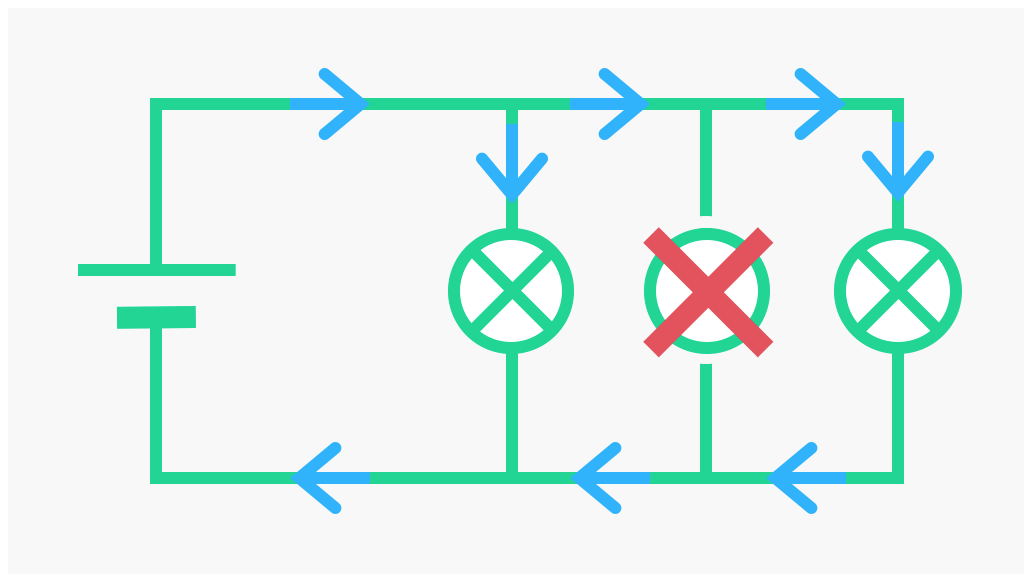Parallel: If one bulb breaks, current can still flow to other bulbs
Quiz

### Congratulations!

10 of 10 questions completed

+ ⭐️ collected.

• You can change your display name at any time.
• at least 1 letter
• at least 1 number
• at least 8 characters